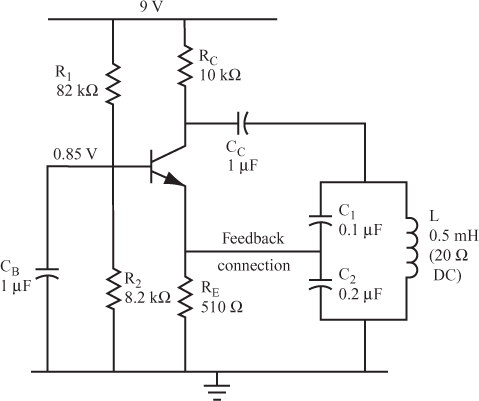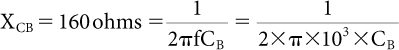# The Colpitts Oscillator

20 Figure 9.15 shows a Colpitts oscillator circuit, the simplest of the LC oscillators to build.

Figure 9.15The feedback signal is taken from the capacitive voltage divider and fed to the emitter. This connection provides a feedback signal to the emitter in the phase required to provide positive feedback.

In this circuit, the reactance of capacitor CB is low enough for the AC signal to pass through it, rather than passing through R2. Capacitor CB should have a reactance, XCB, of less than 160 ohms at the oscillation frequency. If R2 happens to be smaller than 1.6 kΩ, choose a value of XCB that is less than one-tenth of R2.

Question

For the circuit shown in Figure 9.15, what is your first estimate for CB? Assume that fr is equal to 1 kHz, and that Xc equals 160 ohms._____Therefore, CB = 1 μF; larger values of CB also work.

21 Use the Colpitts oscillator component values shown in Figure 9.15 to answer the following questions.

Questions
A. What is the effective total capacitance of the two series capacitors in the tuned circuit?
CT = _____
B. What is the oscillator frequency?
fr = _____
C. What is the impedance of the tuned circuit at this frequency?
Z = _____
D. What fraction of the output voltage is fed back?
Vf = _____
E. What ...

Get Complete Electronics Self-Teaching Guide with Projects now with the O’Reilly learning platform.

O’Reilly members experience live online training, plus books, videos, and digital content from nearly 200 publishers.